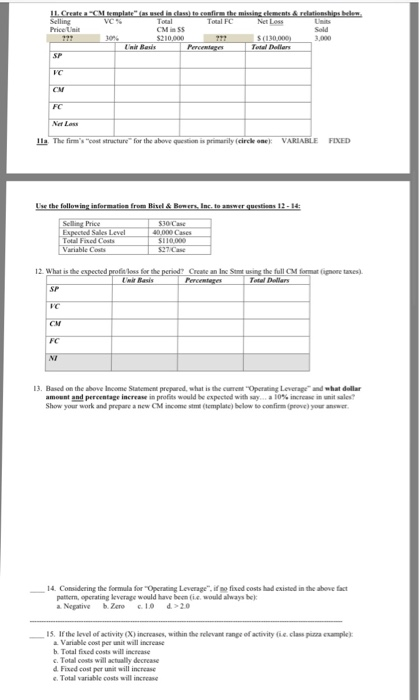# Question & Answer: The firm's "cost structure" for the above question is primarily (circle one: VARIABLE FIXED What is the ex…..The firm’s “cost structure” for the above question is primarily (circle one: VARIABLE FIXED What is the expected profit/loss for the period? Create an Inc Stmt using the full CM format (Ignore taxes). Based on the above Income Statement prepared, what is the current “Operating Leverage” and what dollar amount and percentage increase in profits would be expected with say a 10% increase in unit sales? Show your work and prepare a new CM income stmt (template) below to confirm (prove) your answer. Considering the formula for “Operating Leverage”, if no fixed costs had existed in the above fact pattern, operating leverage would have been (i.e, would always be): a. Negative b. Zero c. 1.0 d. > 2.0 If the level of activity (X) increases, within the relevant range of activity (i.e. class pizza example) a Variable cost per unit will increase b. Total fixed costs will increase C. Total costs will actually decrease d. Fixed cost per unit will increase e. Total variable costs will increase